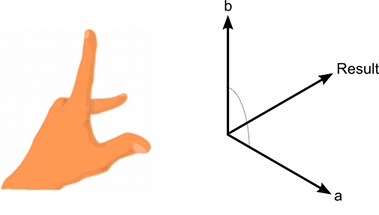Version: 2017.3
Idioma: Español

# Vector3.Cross

Cambiar al Manual
public static Vector3 Cross (Vector3 lhs, Vector3 rhs);

## Descripción

Producto vectorial de dos vectores.

The cross product of two vectors results in a third vector which is perpendicular to the two input vectors. The result's magnitude is equal to the magnitudes of the two inputs multiplied together and then multiplied by the sine of the angle between the inputs. You can determine the direction of the result vector using the "left hand rule".The left hand rule applied to Cross(a, b).

```using UnityEngine;
using System.Collections;public class ExampleClass : MonoBehaviour {
Vector3 GetNormal(Vector3 a, Vector3 b, Vector3 c) {
Vector3 side1 = b - a;
Vector3 side2 = c - a;
return Vector3.Cross(side1, side2).normalized;
}
}
```